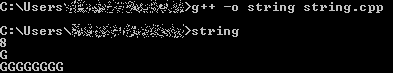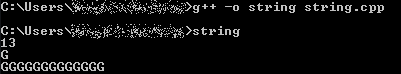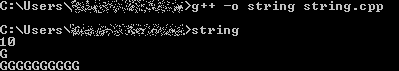Open in App
Not now

# Create a string of specific length in C++

• Difficulty Level : Basic
• Last Updated : 16 Jul, 2021

C++ has in its definition a way to represent a sequence of characters as an object of a class. This class is called std::string. String class stores the characters as a sequence of bytes with the functionality of allowing access to the single-byte characters. This article focuses on discussing how to create a string of specific characters with specific lengths in C++. Let’s have a look at the various methods available to get a specific length string with the specific character where the character and the length of the string are taken as user input.

Method 1: In this method, for loop is used. The idea is to first, get the length L of the string is taken as input and then input the specific character C and initialize empty string str. Now, iterate a loop for L number of times. In every iteration, the specific character is concatenated with string str until the for loop ends.

Below is the implementation of the above approach:

## C++

 `// C++ program for the above approach``#include ``using` `namespace` `std;` `// Driver Code``int` `main()``{``    ``// Initialize the specific length``    ``// and character of string``    ``int` `L;``    ``char` `C;` `    ``// Initialize the empty string``    ``// for concatenation``    ``string str = ``""``;` `    ``// Input the specific length and``    ``// character of string``    ``cin >> L;``    ``cin >> C;` `    ``// Run the for loop L times``    ``for` `(``int` `i = 0; i < L; i++)``        ``str = str + C;` `    ``// Output the result string``    ``cout << str;` `    ``return` `0;``}`

Output:Method 2: In this method, the predefined function append() is used in this method. This function is in the string library in C++. Therefore, the idea is to first initialize the length L, specific character C, and the string str, then input the length of the string that the user wants and the specific character. After that call the append() function with the necessary parameters, and then print the string str.

Below is the implementation of the above approach:

## C++

 `// C++ program for the above approach``#include ``using` `namespace` `std;` `// Driver Code``int` `main()``{``    ``// Initialize the specific length``    ``// and character of string``    ``int` `L;``    ``char` `C;` `    ``// Initialize the string``    ``string str;` `    ``// Input the specific length and``    ``// character of string``    ``cin >> L;``    ``cin >> C;` `    ``// Call the append function with``    ``// specific parameters``    ``str.append(L, C);` `    ``// Output the result string``    ``cout << str;` `    ``return` `0;``}`

Output:Method 3: This method is very easy, fast, and efficient. In this method, the constructor of std::string is being used. Simply input the length L, character C, and initialize the string object with default values.

Below is the implementation of the above approach:

## C++

 `// C++ program for the above approach``#include ``using` `namespace` `std;` `// Driver Code``int` `main()``{``    ``// Initialize the specific length``    ``// and character of string``    ``int` `L;``    ``char` `C;` `    ``// Input the specific length``    ``// and character of string``    ``cin >> L;``    ``cin >> C;` `    ``// Initialize the string object``    ``// with default values``    ``string str(L, C);` `    ``// Output the result string``    ``cout << str;` `    ``return` `0;``}`

Output:My Personal Notes arrow_drop_up